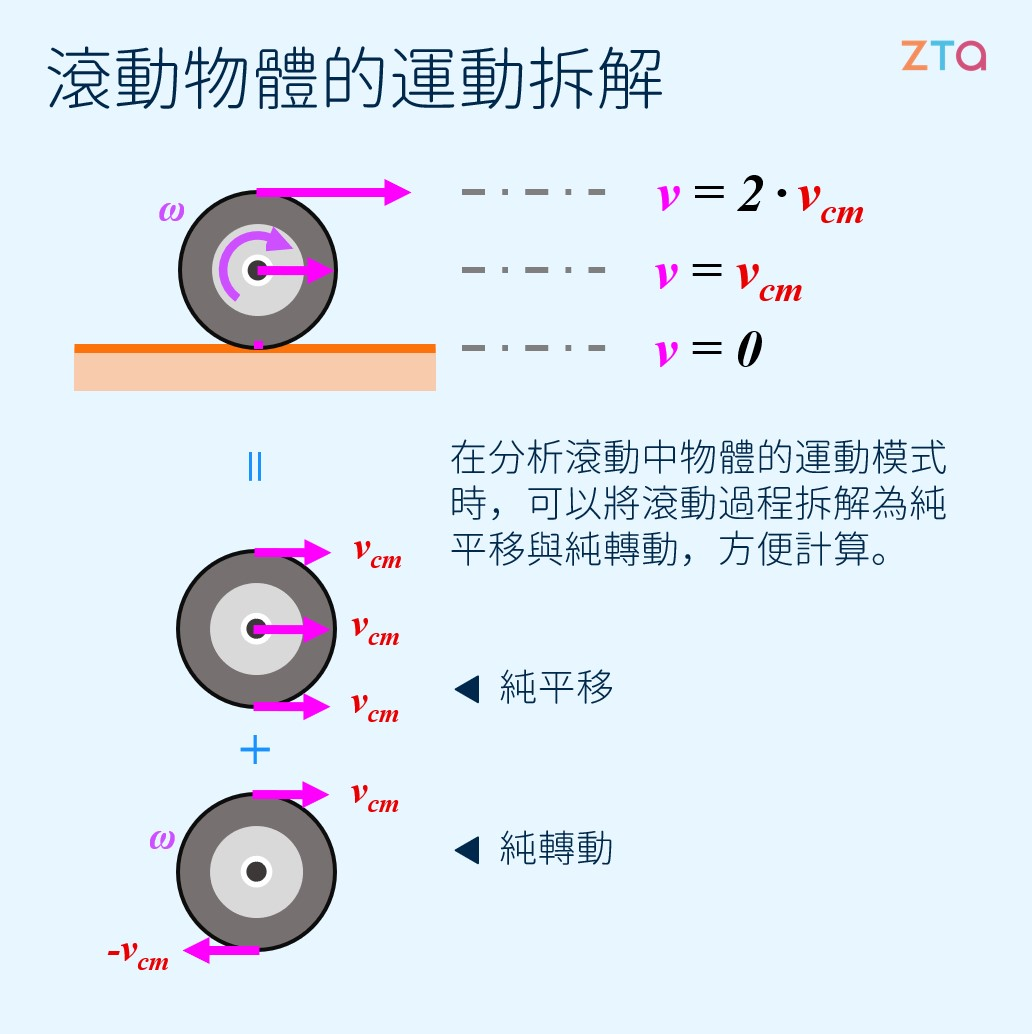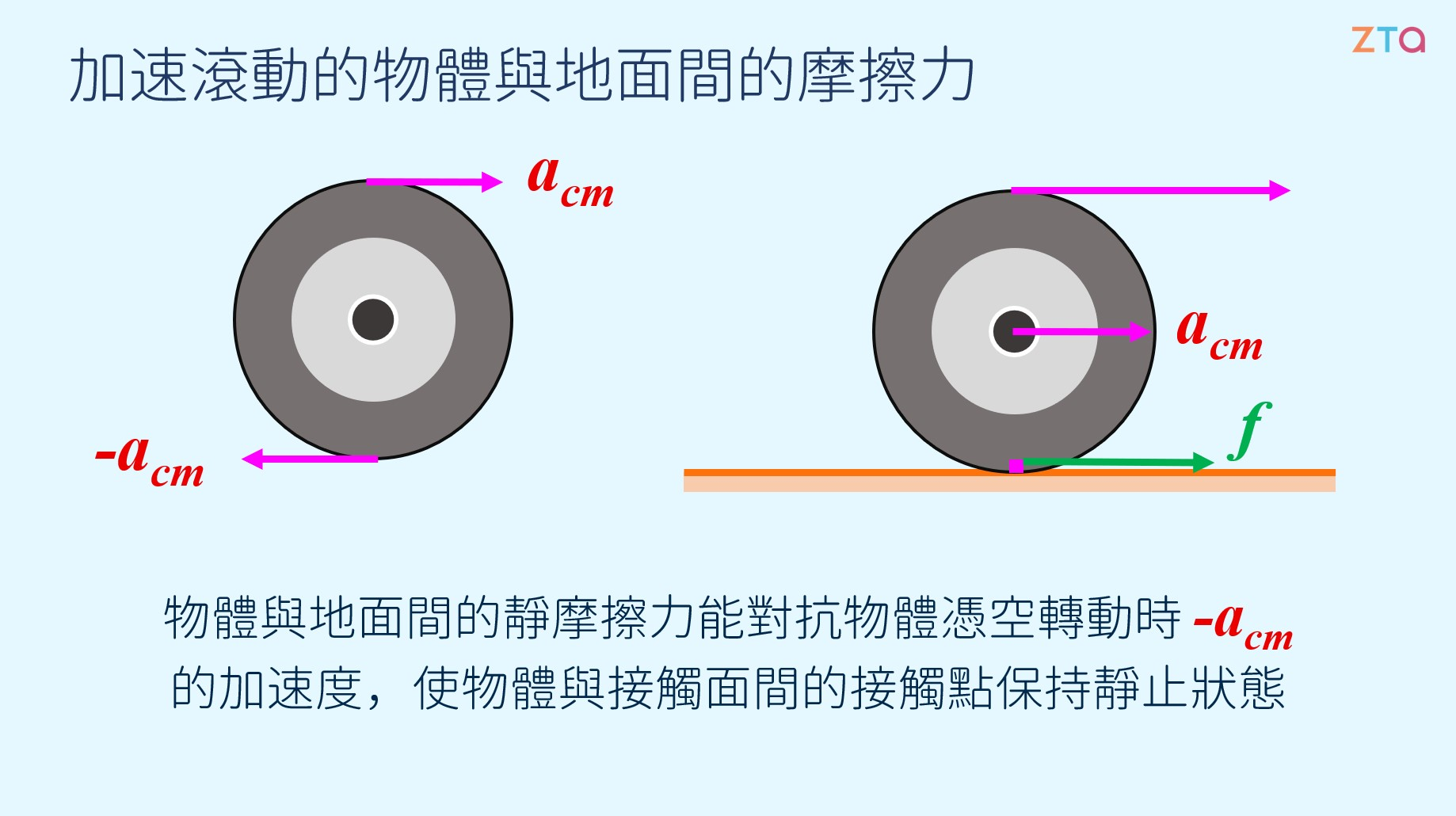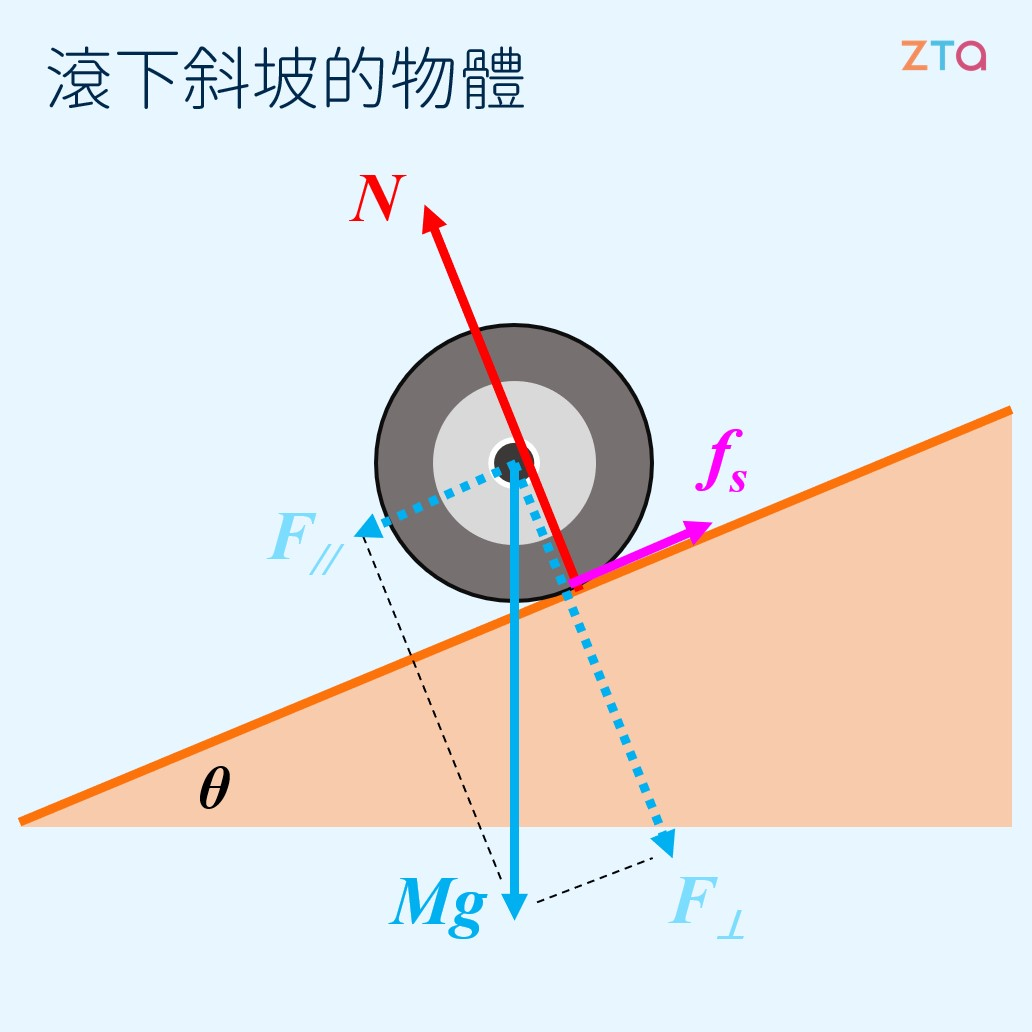# 滾動中的物體

## 滾動 = 轉動 + 平移## 滾動動能

$$E_k = E_v+E_\omega$$$$=\frac{1}{2}I\omega^2+\frac{1}{2}mv^2$$

## 接觸點的摩擦力## 沿著斜面滾動$$Ma_{cm} = f_s - Mg\cdot sin(\theta)$$

$$Rf_s = I_{cm}\alpha$$

$$Rf_s = I_{cm}\frac{-a}{R}$$

$$f_s = I_{cm}\frac{-a}{R^2}$$

$$Ma_{cm} = I_{cm}\frac{-a_{cm}}{R^2} - Mg\cdot sin(\theta)$$

$$a_{cm}(M+\frac{I_{cm}}{R^2}) = -Mg\cdot sin(\theta)$$

$$a_{cm} = \frac{-Mg\cdot sin(\theta)}{M + I_{cm}/R^2}$$$$=\frac{-g\cdot sin(\theta)}{1+I_{cm}/(MR^2)}$$

+1